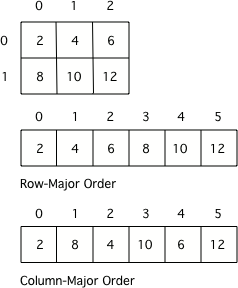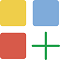# 8.9.2. Picture Lab A4: 2D Arrays in Java¶

In this activity you will work with integer data stored in a two-dimensional array. Some programming languages use a one-dimensional (1D) array to represent a two-dimensional (2D) array with the data in either row-major or column-major order. Row-major order in a 1D array means that all the data for the first row is stored before the data for the next row in the 1D array. Column-major order in a 1D array means that all the data for the first column is stored before the data for the next column in the 1D array. The order matters, because you need to calculate the position in the 1D array based on the order, the number of rows and columns, and the current column and row numbers (indices). The rows and columns are numbered (indexed) and often that numbering starts at 0 as it does in Java. The top left row has an index of 0 and the top left column has an index of 0. The row number (index) increases from top to bottom and the column number (index) increases from left to right.Figure 1: A 2D array stored in row-major order or column-major order as a 1D array.¶

Java actually uses arrays of arrays to represent 2D arrays. This means that each element in the outer array is a reference to another array. The data can be in either row-major or column-major order. The AP Computer Science A course specification tells you to assume that all 2D arrays are row-major, which means that the outer array in Java represents the rows and the inner arrays represent the columns.

To loop through the values in a 2D array you must have two indexes. One index is used to change the row index and one is used to change the column index. You can use nested loops, which is one for loop inside of another, to loop through all the values in a 2D array. Let’s try some nested loops with 2D arrays.

Here is the code for the getTotal() method in the IntArrayWorker class that totals all the values in a 2D array of integers in a private instance variable (field in the class) named matrix. Notice the nested for loop and how it uses matrix.length to get the number of rows and matrix.length to get the number of columns. Since matrix returns the inner array in a 2D array, you can use matrix.length to get the number of columns.

public int getTotal()
{
int total = 0;
for (int row = 0; row < matrix.length; row++)
{
for (int col = 0; col < matrix.length; col++)
{
total = total + matrix[row][col];
}
}
}


Because Java two-dimensional arrays are actually arrays of arrays, you can also get the total using nested for-each loops as shown in getTotalNested below. The outer loop will loop through the outer array (each of the rows) and the inner loop will loop through the inner array (columns in that row). You can use a nested for-each loop whenever you want to loop through all items in a 2D array and you don’t need to know the row index or column index.

public int getTotalNested()
{
int total = 0;
for (int[] rowArray : matrix)
{
for (int item : rowArray)
{
total = total + item;
}
}
}Coding Exercises

1. Write a getCount method in the IntArrayWorker class that returns the count of the number of times a passed integer value is found in the matrix. There is already a method to test this in IntArrayWorkerTester.

Write a getCount method in the IntArrayWorker class that returns the count of the number of times a passed integer value is found in the matrix.

2. Write a getLargest method in the IntArrayWorker class that returns the largest value in the matrix. There is already a method to test this in IntArrayWorkerTester.

Write a getLargest method in the IntArrayWorker class that returns the largest value in the matrix.

3. Write a getColTotal method in the IntArrayWorker class that returns the total of all integers in a specified column. There is already a method to test this in IntArrayWorkerTester.

Write a getColTotal method in the IntArrayWorker class that returns the total of all integers in a specified column.